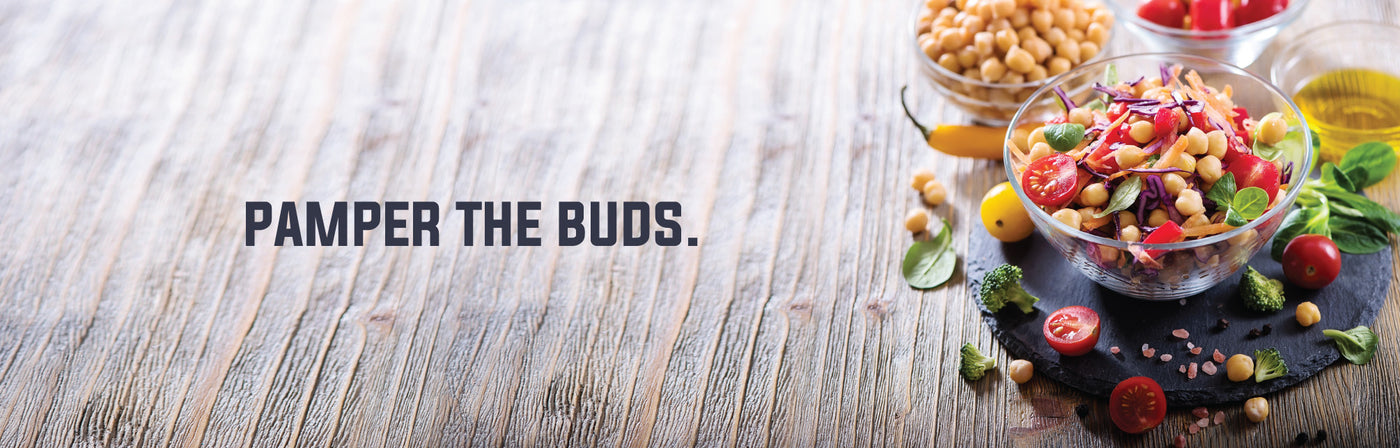Tasty indulgenceSort by:
Rs. 129.00

Rs. 149.00

Rs. 129.00

Rs. 129.00

Rs. 189.00

Rs. 189.00

Rs. 169.00

Rs. 199.00

Rs. 239.00

Rs. 169.00

Rs. 129.00

Rs. 199.00

Rs. 99.00

Rs. 149.00

Rs. 149.00

Rs. 129.00

Rs. 129.00

Rs. 199.00

Rs. 120.00

Rs. 160.00

Rs. 160.00

Rs. 120.00

Rs. 120.00

Rs. 120.00

Rs. 149.00

Rs. 149.00

Rs. 290.00

Rs. 129.00

Rs. 99.00

Rs. 149.00

Rs. 129.00

Rs. 169.00

Rs. 300.00

Rs. 169.00

Rs. 479.00

Rs. 199.00

Rs. 149.00

Rs. 289.00

Rs. 289.00

Rs. 169.00

Rs. 129.00

Rs. 129.00

Rs. 149.00

Rs. 49.00

Rs. 160.00

Rs. 79.00

Rs. 129.00

Rs. 99.00

Sale

Unavailable

Sold Out